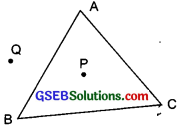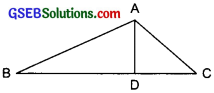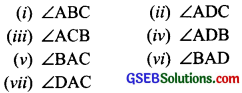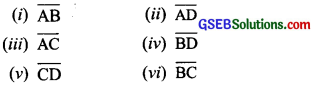# GSEB Solutions Class 6 Maths Chapter 4 Basic Geometrical Ideas Ex 4.4

Gujarat Board GSEB Textbook Solutions Class 6 Maths Chapter 4 Basic Geometrical Ideas Ex 4.4 Textbook Questions and Answers.

## Gujarat Board Textbook Solutions Class 6 Maths Chapter 4 Basic Geometrical Ideas Ex 4.4

Question 1.
Draw a rough sketch of a triangle ABC. Mark a point P in its interior and a point Q in its exterior is the point A in its exterior or in its interior?
Solution:
Figure given represents a triangle.(a) The point P is in the interior of AABC.
(b) The point Q is in the exterior of the triangle.
(c) No, the point A is neither in its interior nor in its exterior.Question 2.
(a) identify three triangles in the figure.(b) Write the names of seven angles.
(c) Write the names of six line segments.
(d) Which two triangles have $$\angle B$$ as common?
Solution:
(a) Three triangles in the given figure are: ∆ABC, ∆ABD, ∆ADC

(b) Seven angles in the given figure are:(c) Six line segments in the given figure are:(d) In the given figure, ∆ABD and ∆ABC have $$\angle B$$ is common.